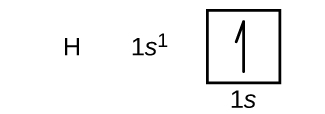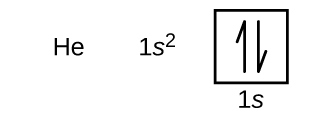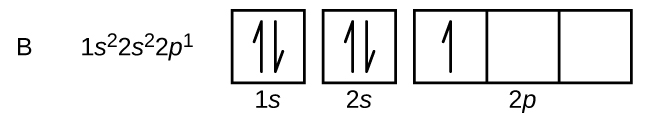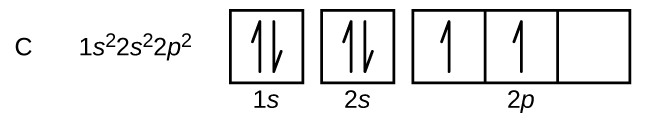# 6.4 Electronic structure of atoms (electron configurations)  (Page 3/15)

 Page 3 / 15Following hydrogen is the noble gas helium, which has an atomic number of 2. The helium atom contains two protons and two electrons. The first electron has the same four quantum numbers as the hydrogen atom electron ( n = 1, l = 0, m l = 0, ${m}_{s}=+\frac{1}{2}$ ). The second electron also goes into the 1 s orbital and fills that orbital. The second electron has the same n , l , and m l quantum numbers, but must have the opposite spin quantum number, ${m}_{s}=-\frac{1}{2}.$ This is in accord with the Pauli exclusion principle: No two electrons in the same atom can have the same set of four quantum numbers. For orbital diagrams, this means two arrows go in each box (representing two electrons in each orbital) and the arrows must point in opposite directions (representing paired spins). The electron configuration and orbital diagram of helium are:The n = 1 shell is completely filled in a helium atom.

The next atom is the alkali metal lithium with an atomic number of 3. The first two electrons in lithium fill the 1 s orbital and have the same sets of four quantum numbers as the two electrons in helium. The remaining electron must occupy the orbital of next lowest energy, the 2 s orbital ( [link] or [link] ). Thus, the electron configuration and orbital diagram of lithium are:An atom of the alkaline earth metal beryllium, with an atomic number of 4, contains four protons in the nucleus and four electrons surrounding the nucleus. The fourth electron fills the remaining space in the 2 s orbital.An atom of boron (atomic number 5) contains five electrons. The n = 1 shell is filled with two electrons and three electrons will occupy the n = 2 shell. Because any s subshell can contain only two electrons, the fifth electron must occupy the next energy level, which will be a 2 p orbital. There are three degenerate 2 p orbitals ( m l = −1, 0, +1) and the electron can occupy any one of these p orbitals. When drawing orbital diagrams, we include empty boxes to depict any empty orbitals in the same subshell that we are filling.Carbon (atomic number 6) has six electrons. Four of them fill the 1 s and 2 s orbitals. The remaining two electrons occupy the 2 p subshell. We now have a choice of filling one of the 2 p orbitals and pairing the electrons or of leaving the electrons unpaired in two different, but degenerate, p orbitals. The orbitals are filled as described by Hund’s rule    : the lowest-energy configuration for an atom with electrons within a set of degenerate orbitals is that having the maximum number of unpaired electrons. Thus, the two electrons in the carbon 2 p orbitals have identical n , l , and m s quantum numbers and differ in their m l quantum number (in accord with the Pauli exclusion principle). The electron configuration and orbital diagram for carbon are:Nitrogen (atomic number 7) fills the 1 s and 2 s subshells and has one electron in each of the three 2 p orbitals, in accordance with Hund’s rule. These three electrons have unpaired spins. Oxygen (atomic number 8) has a pair of electrons in any one of the 2 p orbitals (the electrons have opposite spins) and a single electron in each of the other two. Fluorine (atomic number 9) has only one 2 p orbital containing an unpaired electron. All of the electrons in the noble gas neon (atomic number 10) are paired, and all of the orbitals in the n = 1 and the n = 2 shells are filled. The electron configurations and orbital diagrams of these four elements are:

what are oxidation numbers
pls what is electrolysis
Electrolysis is the process by which ionic substances are decomposed (broken down) into simpler substances when an electric current is passed through them. ... Electricity is the flow of electrons or ions. For electrolysis to work, the compound must contain ions.
AZEEZ
thanks
Idowu
what is the basicity of an atom
basicity is the number of replaceable Hydrogen atoms in a Molecule. in H2SO4, the basicity is 2. in Hcl, the basicity is 1
Inemesit
how to solve oxidation number
mention some examples of ester
do you mean ether?
Megan
what do converging lines on a mass Spectra represent
would I do to help me know this topic ?
Bulus
oi
Amargo
what the physic?
who is albert heistein?
Bassidi
similarities between elements in the same group and period
what is the ratio of hydrogen to oxulygen in carbohydrates
bunubyyvyhinuvgtvbjnjnygtcrc
yvcrzezalakhhehuzhbshsunakakoaak
what is poh and ph
please what is the chemical configuration of sodium
Sharon
2.8.1
david
1s²2s²2p⁶3s¹
Haile
2, 6, 2, 1
Salman
1s2, 2s2, 2px2, 2py2, 2pz2, 3s1
Justice
1s2,2s2,2py2,2
Maryify
1s2,2s2,2p6,
Francis
1s2,2s2,2px2,2py2,2pz2,3s1
Nnyila
what is criteria purity
cathode is a negative ion why is it that u said is negative
cathode is a negative electrode while cation is a positive ion. cation move towards cathode plate.
king
CH3COOH +NaOH ,complete the equation
compare and contrast the electrical conductivity of HCl and CH3cooH
The must be in dissolved in water (aqueous). Electrical conductivity is measured in Siemens (s). HCl (aq) has higher conductivity, as it fully ionises (small portion of CH3COOH (aq) ionises) when dissolved in water. Thus, more free ions to carry charge.
Abdelkarim
HCl being an strong acid will fully ionize in water thus producing more mobile ions for electrical conduction than the carboxylic acid
Valentine
differiante between a weak and a strong acid
david
how can I tell when an acid is weak or Strong
Amarachi
an aqueous solution of copper sulphate was electrolysed between graphite electrodes. state what was observed at the cathode
write the equation for the reaction that took place at the anode
Bakanya
what is enthalpy of combustion
Bakanya
Enthalpy change of combustion: It is the enthalpy change when 1 mole of substance is combusted with excess oxygen under standard conditions. Elements are in their standard states. Conditions: pressure = 1 atm Temperature =25°C
Abdelkarim
Observation at Cathode: Cu metal deposit (pink/red solid).
Abdelkarim
Equation at Anode: (SO4)^2- + 4H^+ + 2e^- __> SO2 + 2H2O
Abdelkarim
Equation : CuSO4 -> Cu^2+ + SO4^2- equation at katode: 2Cu^2+ + 4e -> 2Cu equation at anode: 2H2O -> 4H+ + O2 +4e at the anode which reacts is water because SO4 ^ 2- cannot be electrolyzed in the anode
NikenByByBy David GeltnerBy OpenStaxBy Cameron CaseyBy OpenStaxBy Jemekia WeedenBy Bonnie HurstBy Madison ChristianBy Anonymous UserBy Christine ZeelieBy Madison Christian